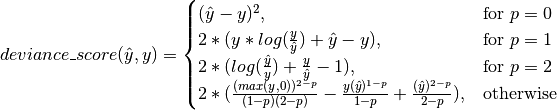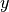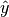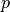Shortcuts

# Tweedie Deviance Score¶

## Module Interface¶

class torchmetrics.TweedieDevianceScore(power=0.0, **kwargs)[source]

Computes the Tweedie Deviance Score between targets and predictions:whereis a tensor of targets values,is a tensor of predictions, andis the power.

As input to forward and update the metric accepts the following input:

• preds (Tensor): Predicted float tensor with shape (N,...)

• target (Tensor): Ground truth float tensor with shape (N,...)

As output of forward and compute the metric returns the following output:

Parameters
• power (float) –

• power < 0 : Extreme stable distribution. (Requires: preds > 0.)

• power = 0 : Normal distribution. (Requires: targets and preds can be any real numbers.)

• power = 1 : Poisson distribution. (Requires: targets >= 0 and y_pred > 0.)

• 1 < p < 2 : Compound Poisson distribution. (Requires: targets >= 0 and preds > 0.)

• power = 2 : Gamma distribution. (Requires: targets > 0 and preds > 0.)

• power = 3 : Inverse Gaussian distribution. (Requires: targets > 0 and preds > 0.)

• otherwise : Positive stable distribution. (Requires: targets > 0 and preds > 0.)

• kwargs (Any) – Additional keyword arguments, see Advanced metric settings for more info.

Example

>>> from torchmetrics import TweedieDevianceScore
>>> targets = torch.tensor([1.0, 2.0, 3.0, 4.0])
>>> preds = torch.tensor([4.0, 3.0, 2.0, 1.0])
>>> deviance_score = TweedieDevianceScore(power=2)
>>> deviance_score(preds, targets)
tensor(1.2083)


Initializes internal Module state, shared by both nn.Module and ScriptModule.

## Functional Interface¶

torchmetrics.functional.tweedie_deviance_score(preds, targets, power=0.0)[source]

Computes the Tweedie Deviance Score between targets and predictions:whereis a tensor of targets values,is a tensor of predictions, andis the power.

Parameters
• preds (Tensor) – Predicted tensor with shape (N,...)

• targets (Tensor) – Ground truth tensor with shape (N,...)

• power (float) –

• power < 0 : Extreme stable distribution. (Requires: preds > 0.)

• power = 0 : Normal distribution. (Requires: targets and preds can be any real numbers.)

• power = 1 : Poisson distribution. (Requires: targets >= 0 and y_pred > 0.)

• 1 < p < 2 : Compound Poisson distribution. (Requires: targets >= 0 and preds > 0.)

• power = 2 : Gamma distribution. (Requires: targets > 0 and preds > 0.)

• power = 3 : Inverse Gaussian distribution. (Requires: targets > 0 and preds > 0.)

• otherwise : Positive stable distribution. (Requires: targets > 0 and preds > 0.)

Example

>>> from torchmetrics.functional import tweedie_deviance_score
>>> targets = torch.tensor([1.0, 2.0, 3.0, 4.0])
>>> preds = torch.tensor([4.0, 3.0, 2.0, 1.0])
>>> tweedie_deviance_score(preds, targets, power=2)
tensor(1.2083)

Return type

Tensor

© Copyright Copyright (c) 2020-2023, Lightning-AI et al... Revision 825d17f3.

Built with Sphinx using a theme provided by Read the Docs.
Versions
latest
stable
v0.11.3
v0.11.2
v0.11.1
v0.11.0
v0.10.3
v0.10.2
v0.10.1
v0.10.0
v0.9.3
v0.9.2
v0.9.1
v0.9.0
v0.8.2
v0.8.1
v0.8.0
v0.7.3
v0.7.2
v0.7.1
v0.7.0
v0.6.2
v0.6.1
v0.6.0
v0.5.1
v0.5.0
v0.4.1
v0.4.0
v0.3.2
v0.3.1
v0.3.0
v0.2.0
v0.1.0